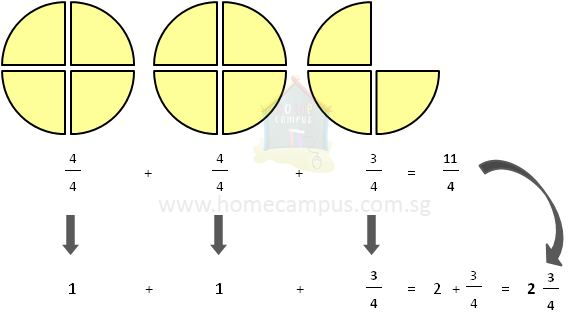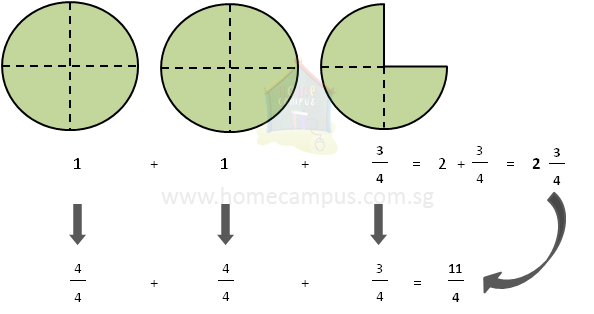## Improper Fractions and Mixed Numbers

Practice Unlimited Questions

#### What is an improper fraction?

An improper fraction is a fraction in which the numerator is bigger than or equal to the denominator.

#### What is a mixed number?

A mixed number is made up of two parts - a whole number and a proper fraction.

#### 1. The video below explains what improper fractions and mixed numbers are.

Improper fractions and mixed numbers are 2 different ways to represent the same number.

Therefore, improper fractions can be converted to mixed numbers and mixed numbers can be converted back to improper fractions.
To write an improper fraction, you count the pizza slices.

To write a mixed number, you count the whole pizzas.
Denominator means how many slices to cut each pizza into.

Numerator means how many slices you get.

#### 2. Convert    11 4 to a mixed number.

Let's represent the improper fraction
 11 4
using pizza slices.

The denominator 4 means that each pizza should be cut into 4 slices.
The numerator 11 means that your share of the pizza is 11 slices.To convert an improper fraction to a mixed number, divide the numerator by the denominator (11 divided by 4).

 11 4

=  11  ÷  4
2 R 3   (2 whole pizzas and 3 slices out of 4 of another pizza)
 2 3 4

#### 3. Convert       2    3    4   to an improper fraction.

Let's represent the mixed fraction
 2 3 4
using pizza slices.

The whole number part 2 means there are 2 whole pizzas.

The fractional part
 3 4
means there are 3 slices out of 4 of another pizza.

Converting a mixed number to an improper fraction means you are given whole pizzas and you have to find out how many pizza slices they will make.To convert a mixed number to an improper fraction, first, multiply the whole number part by the denominator of the fractional part, then add the numerator to the product.

 2 3 4

=   2  +
 3 4

 (2 x 4) + 3 4

 8 + 3 4

 11 4
If each pizza has got 4 slices then 2 whole pizzas will have 2 × 4 slices or 8 slices.